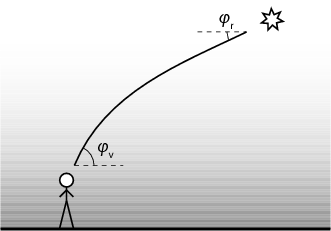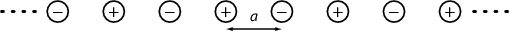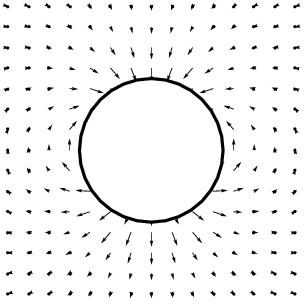5th of November, 2008

1. Show that the retarded potentials $\begin{array}{llll}\hfill V\left(\stackrel{\to }{r},t\right)& =\frac{1}{4\pi {\epsilon }_{0}}\int \frac{\rho \left({\stackrel{\to }{r}}^{\prime },{t}_{r}\right)}{R}\phantom{\rule{3.26212pt}{0ex}}{d}^{3}{\stackrel{\to }{r}}^{\prime }\phantom{\rule{2em}{0ex}}& \hfill & \phantom{\rule{2em}{0ex}}\\ \hfill \stackrel{\to }{A}\left(\stackrel{\to }{r},t\right)& =\frac{{\mu }_{0}}{4\pi }\int \frac{\stackrel{\to }{j}\left({\stackrel{\to }{r}}^{\prime },{t}_{r}\right)}{R}\phantom{\rule{3.26212pt}{0ex}}{d}^{3}{\stackrel{\to }{r}}^{\prime },\phantom{\rule{2em}{0ex}}& \hfill & \phantom{\rule{2em}{0ex}}\end{array}$

where $\stackrel{\to }{R}=\stackrel{\to }{r}-{\stackrel{\to }{r}}^{\prime }$ and ${t}_{r}=t-R∕c$, satisfy the Lorenz gauge condition:

$\nabla \cdot \stackrel{\to }{A}+\frac{1}{{c}^{2}}\frac{\partial V}{\partial t}=0.$

(Note: ${d}^{3}{\stackrel{\to }{r}}^{\prime }$ denotes volume integration with respect to the vector ${\stackrel{\to }{r}}^{\prime }$.)

1. Show that in a static electric field a point charge cannot have a stable equilibrium point in vacuum!
2. Show that in a static magnetic field a tiny magnet cannot have a stable equilibrium point in vacuum. First solve the problem in the simpler case when the spatial orientation of the magnet is fixed (i.e. its magnetic moment . Then solve the problem in the general case when the magnet can rotate freely.
2. A star is visible at altitude ${\phi }_{v}$. The index of refraction of air is $n\left(h\right)$ as a function of height above the ground. What is the real altitude (${\phi }_{r}$) of the star (correcting for atmospheric refraction) if it is high enough so that the curvature of the earth can be ignored? (Consider the Earth flat.)3. A plasma is an ionized gas, containing positively charged ions and negatively charges electrons.

An electromagnetic wave is travelling through a plasma, which contains $n$ electrons per unit volume. The electrons are brought into motion by the electric field, but the ions, which have a much lower specific charge, can be safely considered stationary.

Calculate the relative permittivity ${\epsilon }_{r}$ of the plasma as a function of the angular frequency $\omega$ of the electromagnetic wave.

4. What is the electrostatic energy per atom of a linear ionic crystal?

Hint: The Taylor expansion of the logarithm function will prove helpful.Figure 1: A linear lattice of $+$ and $-$ ions. The lattice constant is $a$.

5. A ball is falling through water with steady speed. Calculate the velocity field of water (the velocity of flow at every point in space: $\stackrel{\to }{v}\left(\stackrel{\to }{r}\right)$)! (See fig. 2.)

Assume that water is incompressible (its density is constant), and that the flow is irrotational ($\nabla ×\stackrel{\to }{v}=0$). These assumptions are rarely correct for real water, however, if the ball is falling neither “too fast” (there is no turbulence), nor “too slow” (the viscous forces are negligible), then we can get an approximation about how the water is flowing around the ball.

Hint: There is an electrostatic analogue for the equations!Figure 2: Estimate of the flow field around a ball falling downwards.

Back to the main page